Problem Set 1---Due February 4

1)      Consider a quantum system composed of two subsystems.  Each subsystem is expressed in terms of a basis of orthonormal states which are eigenstates of some hermitian operator (associated with some physical properties).  A basis for the combined system can be given by states of the form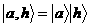where the first label denotes system 1 and the second system 2.  Suppose the state of the system is given by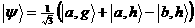.

a)      Show that the state is properly normalized.

b)      Suppose both system 1 and 2 are measured.  What is the probability that we will find the system in the state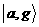?

c)      Suppose system 2 is measured and found to be in state. Given this, what is the probability that system 1 will be in state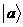?

d)     Suppose system 2 is measured and found to be in state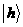. Given this, what is the probability that system 1 will be in state?

2)      In class we considered the two dimensional isotropic harmonic oscillator (where isotropic means the spring constant is the same in all directions.  We showed the energy spectrum was given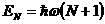with the Nth level having degeneracy of (N+1).  In this problem, generalize the analysis to three dimensions: show that for a three dimensional isotropic harmonic oscillator the showed the energy spectrum was given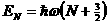with the Nth level having degeneracy of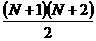.

3)      Consider two distinct systems with two degrees of freedom.  The first one is a system of  two particles (of masses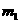and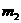) confined to a one-dimensional box of length L.  The second is a single particle (of mass m) confined to a two-dimensional box with sides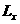and.  Show that the two systems have exactly the same energy spectrum if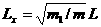and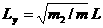.

4)      In class we discussed the separation of variables for the three dimensional time-independent Schrödinger equation with a spherically symmetric potential.  In this problem I want you to work thorough the equivalent for a two dimension system with an axially symmetric potential.  In particular consider the equationin polar coordinates.  Show that the it has solutions of the form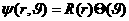with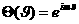(integer m) and R satisfying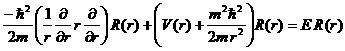.